Next: Additional remarks Up: Notions of relativistic dynamics Previous: Energy and momentum in SRT   Contents

## The Maxwell equations

The following brief comment concerns the Maxwell equations (their conventional present form). Recall that they were obtained by generalization of experimental facts (phenomenologically) at low velocities (by analogy with the hydrodynamics). Therefore, it should not be expected that these equations were guessed in the final form. Maxwell's equations (or the wave equation) define the phase velocity, whereas the theory of relativity "pretends" to the maximum signal velocity (a group velocity). Actually, since some specific light is used always, the quantity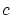must be marked off some index: instead ofwe can write the parametric dependence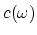, and the wave equation will then be the equation for the Fourier-harmonics. Since modern apologists of relativism abandoned the visualization and the principal necessity of medium's models for the light propagation, the way of generalization of Maxwell's equation becomes not uniquely defined even for the "absolute emptiness" in the case of non-monochromatic light, not to mention the passage to real nonlinear madia (including properties of "intermolecular emptiness", mechanisms of absorption and the light reradiation by moleculs etc.). From pure mathematical considerations and without physical principles, such generalizations can be introduced as much as you desire, and all of them are equal in rights. The requirement that the Maxwell equations be invariant with respect to transformations of coordinates and time is rather vacillating, since if only the measured effects of these fields correspond to the values really observed in the experiment, then the fields and equations for them can be introduced in many ways. So, for instance, it was shown in  that there exist non-local transformations of fields which retain the Maxwell equations with invariable time. It was shown in  that non-linear and non-local transformations can be introduced, so that for some particular transformations of fields, the field equations are invariant with respect to the Galilean transformations.

Let us demonstrate the methodological contradiction of generally-accepted transformations for the fields. Let there be two infinite non-charged parallel wires. Let the electrons in both wires move in the same direction at constant speed relative to a positively charged frame (i.e. we have equal current densities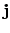). Then for the classical case, in the expression for the field the quantity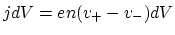is an invariant, i.e. the field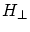and the effect of this field do not depend on the velocity of the system. But for the relativistic consideration (since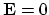) we have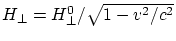, i.e. this field depends on the speed of motion of the observer. However, the following two cases are obviously equivalent:
(1) the system with velocity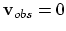, i.e. the observer is resting relative to the frame, and the electrons are moving at velocity, and
(2) the system is moving at velocity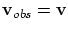, i.e. the observer is resting relative to the electrons, and the frame (with positive ions) is moving in the opposite direction at velocity(the same current). But the relativistic formula gives for these cases different values of(and effects of fields), which is absurd. Besides, the SRT description of transitions from an inertial system to another ones becomes fully inconsistent for the three-dimensional case with non-neutral currents (with beams of charged particles, for example).

Now we shall analyze the "principal" question on the invariance of the Maxwell equations, which is widely advertised in SRT. The invariance of the Maxwell equations with respect to the Lorentz transformations implies nothing for the other phenomena. First, the Maxwell equations are the equations for fields in the empty space. In such a space we can cut off a half of a segment and increase it as much as twice - then we obtain the same segment. Therefore, in the empty mathematical space one can make use of any frames of references, of self-consistent geometries and conversion factors. All these operations can be determined by the convenience of mathematical description only. However, we can not simply cut-through a living organism and increase it twice under a microscope - the organism will be dead. The presence of real physical bodies and fields in the space specifies natural reference points ("bench-marks"), characteristic scales and interrelations between the objects. All this determines the distinctions of a real physical space from the empty mathematical space. Second, the property of some interactions to propagate in vacuum at the speed of light does not determine the rate of interactions' propagation in a medium. In spite of a drastic role of electromagnetic interactions, the disturbances in media propagate at the speed of sound. From one vacuum-related constantit is impossible to determine (for our "electromagnetic" world) the speeds of sound and light in gases, liquids and solid bodies. It is not clear, how the anisotropy of real solid bodies could arise in the isotropic space. All these and many other properties escape the limits of applicability of the Maxwell equations in the emptiness (the SRT, contrary, prescribes "cloning properties of emptiness" on all properties of material bodies and mediums). Therefore, the fitting of the properties of the entire world under the invariance of the Maxwell equations in the emptiness is too excessive claim of SRT. Third, the partition of a single (in its effect) field into electrical and magnetic parts is rather conventional and, to a considerable extent, arbitrary. Hence, the invariance of these, artificially singled out parts can not have crucial significance. The presence of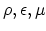coefficients (which depend on coordinates, time, properties of light, etc.) for the Maxwell equations in a medium makes these equations non-invariant relative to the Lorentz transformations (or is it necessary to cancel the objectivity of characteristics of media again?).Next: Additional remarks Up: Notions of relativistic dynamics Previous: Energy and momentum in SRT   Contents
Sergey N. Arteha